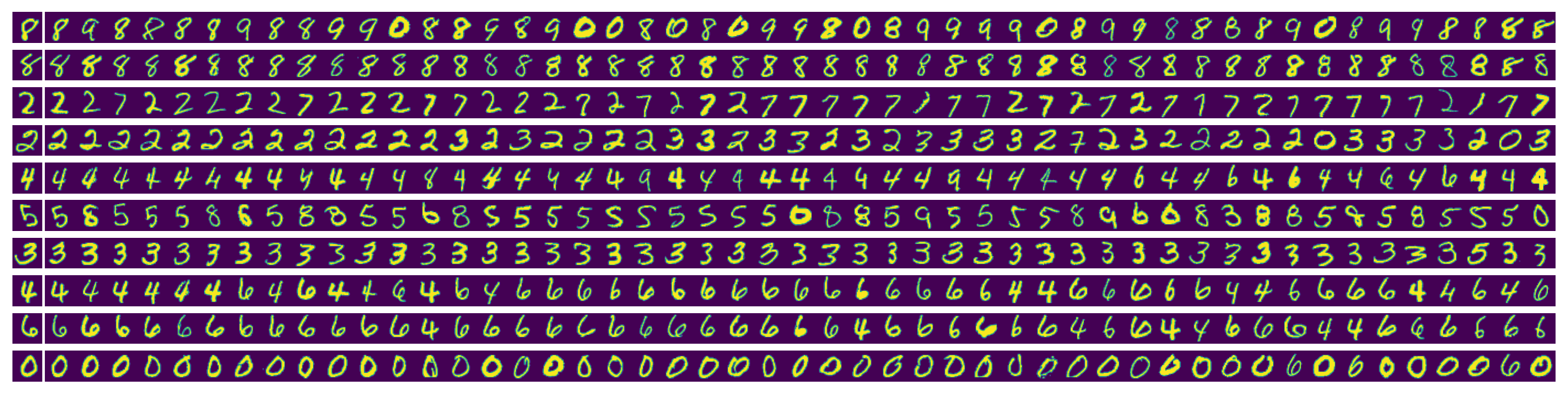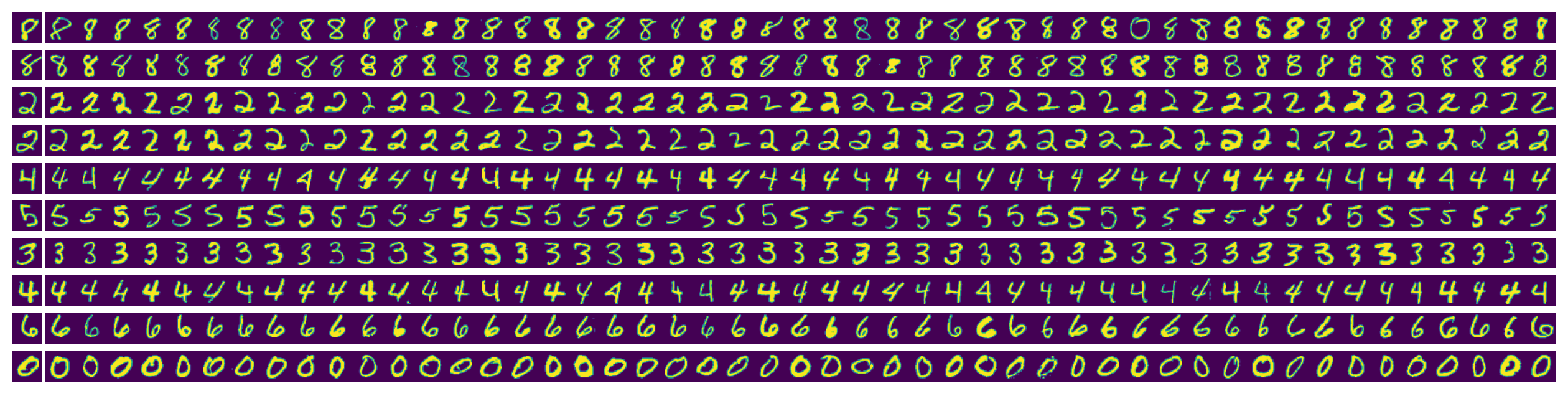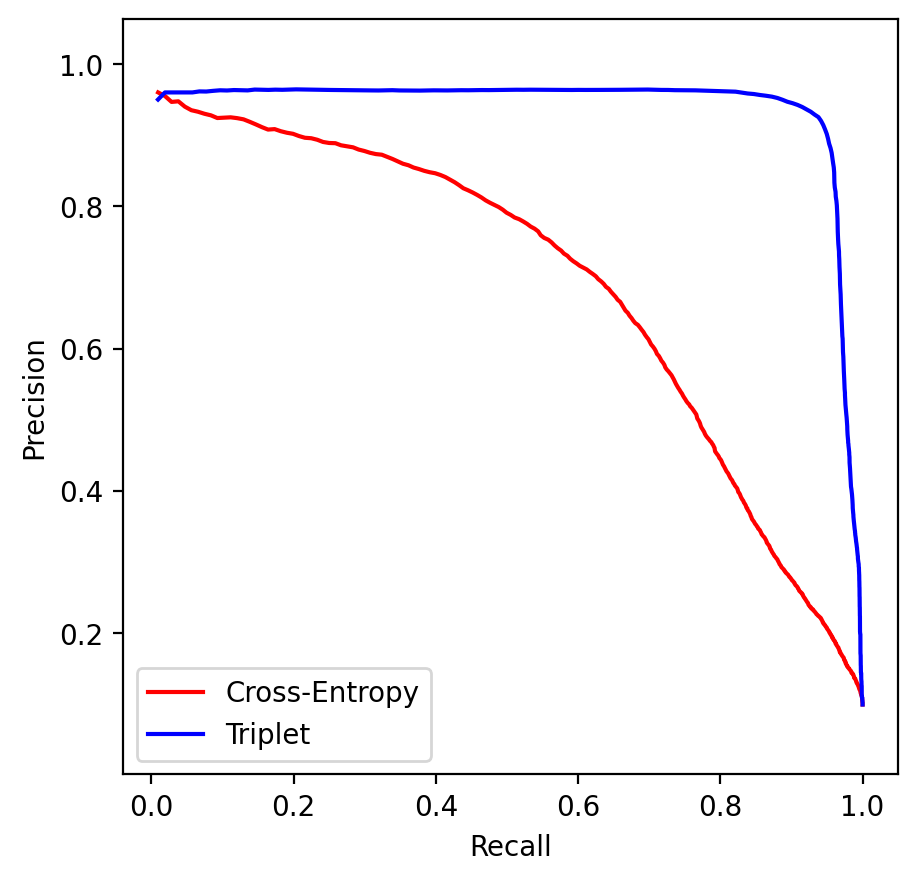Quick links: Schedule | Forum | BRUTE | Lectures | Labs

# Lab 6: Metric Learning

Image retrieval, mean average precision, training embeddings (representations) with triplet loss.

### Templates

Use the provided template. The package contains:

• tools.py — tools for working with dataset.
• triplet.py — template to start with.
• view.ipynb — template for visualization of results.
• ./models/* — pretrained models.

### Introduction

In this lab we want to use a neural network to produce an embedding of images $x$ as feature vectors $f \in \mathbb{R}^d$. These feature vectors can be used then to retrieve similar images by finding the closest neighbors in the embedding space.

### Part 1. Retrieval (4p)

We will use the MNIST dataset and start with a network trained for classification. We will use its last hidden layer representation as the embedding. The network is a small convolutional network defined as follows

class ConvNet(nn.Sequential):
def __init__(self, num_classes: int = 10) -> None:
layers = []
layers += [nn.Conv2d(1, 32, kernel_size=3)]
layers += [nn.ReLU(inplace=True)]
layers += [nn.MaxPool2d(kernel_size=2, stride=2)]
layers += [nn.Conv2d(32, 32, kernel_size=3)]
layers += [nn.ReLU(inplace=True)]
layers += [nn.MaxPool2d(kernel_size=2, stride=2)]
layers += [nn.Conv2d(32, 64, kernel_size=3)]
layers += [nn.ReLU(inplace=True)]
layers += [nn.Flatten()]
layers += [nn.Linear(64 * 2 * 2, num_classes)]
super().__init__(*layers)
self.layers = layers

def features(self, x):
f = nn.Sequential(*self.layers[:-1]).forward(x)
f = nn.functional.normalize(f, p=2, dim=1)
return f
It was trained for classification and achieves $99\%$ accuracy.

1. Implement image retrieval following the iPython notebook template. The template loads the test set and a pretrained model. The pretrained model has method features(x) which given an input batch of samples $x$ outputs a batch of normalized feature vectors $f$, i.e. $\|f_{i,:}\|_2 = 1$ for all $i$. Your task is to retrieve the 50 closest images in the test set for each query image.
Implement a function computing the squared Euclidean distance from the query feature vector to feature vectors of all images in the test set without using loops. Use the test set provided in the template (it contains about 100 test examples of each class).
Hint: Exploit the fact that the feature vectors are normalized and simplify the computation of the squared Euclidean distance & learn how to use 'torch.einsum' function. Alternatively the 'torch.cdist' function may be useful as well.
Display the retrieved images as in the sample result below, where the first image in each row is the query:2. Compute the Mean Average Precision (mAP). You will need the following notions. For each query we sort all items in the dataset by increasing distance from the query. For computing mAP, the query itself should be excluded from the set of retrieved items. Let the relevance ${\rm rel}(i)$ be 1 if the item at position $i$ in this list is of the same class as the query and $0$ otherwise. If we consider the first $k$ items in the list, the precision of the retrieval system at $k$ is defined as $${\rm prec}(k) = \frac{\sum_{i=1}^k {\rm rel}(i)}{k},$$ i.e. the proportion of relevant items in the list of $k$ retrieved items. The recall of the retrieval system at $k$ is defined as $${\rm recall}(k) = \frac{\sum_{i=1}^k {\rm rel}(i)}{T},$$ the ratio of relevant items in the list of length $k$ to the total number of relevant items in the dataset $T$ (images of the same class as query). The average precision is defined as the area under the precision-recall curve which can be computed as $${\rm} AP = \sum_{k=1}^N{\rm prec}(k) \Delta {\rm recall}(k) = \sum_{k=1}^N{\rm prec}(k) \frac{{\rm rel}(k)}{T}.$$ See also wikipedia. Note that $N$ means all examples in the dataset, i.e. we do not limit it to 50 best as in Part 1. The Mean Average Precision (mAP) is the mean of $AP$ over random queries.

Your task is to implement a function evaluate_AP (avoid using loops) which computes the average precision given distances from a query to all other data points and their labels. Using this function, implement evaluate_mAP(net, dataset) which computes the mean average precision using the first 100 (random) dataset points as queries, as provided in the template. The expected result for the classification network is ${\rm mAP} = 0.74$. Plot the precision - recall curve.

Report and discuss: method used to compute all Euclidean distances, figure with retrieved images from 1, precision-recall curve and mAP.

### Part 2. Metric Learning (6p)

In this part we will learn the embedding to directly facilitate the retrieval performance by training with triplet loss. We will use exactly the same network architecture as in Part 1 but train it differently. For an anchor (query) image $a$ let the positive example $p$ be of the same class and a negative example $n$ be of different class than $a$. We want each positive example to be closer to the anchor than all negative examples with a margin $\alpha \geq 0$: $$d(f(a),f(p)) \leq d(f(a),f(n)) - \alpha \ \ \ \forall p,n.$$ The constraint is violated if $d(f(a),f(p)) - d(f(a),f(n)) + \alpha \geq 0$. Accordingly we define the triplet loss as the total violation of these constraints: $$l(a) = \sum_{p,n} \max(d(f(a),f(p)) - d(f(a),f(n)) + \alpha, 0).$$ As function $d$ use the squared Euclidean distance $d(x,y) = \| x-y\|_2^2$ and for alpha select a value in the range $(0,2)$, for example $0.5$. We need to incorporate this loss in the stochastic optimization with mini-batches. Your task is as follows:

1. Implement the function triplet_loss that given a batch of data points represented by their feature vectors and class labels, does the following. The first 10 entries in the batch are considered as anchors. For each anchor $a$ compute the total loss $l(a)$ where $n,p$ go over all possible valid choices in the given batch. Avoid loops in your implementation (with the exception of the loop over anchors $a$).
2. Implement the function train_triplets that performs SGD using batches of data and the triplet loss defined above. Batches of images are sampled in the standard way (images are drawn at random without replacement). Train the model on a GPU server for 100 epochs. Print the total triplet loss in the end of each epoch. Save the training history and the trained network.
3. Compare the t-SNE embeddings of the two networks. Beware of various interpretation pitfalls!

Report and discuss: Optimization settings, plot of the training progress using the saved history. Evaluation of the trained network as in Part 1 (examples of retrieval and mAP score). t-SNE comparison. Precision-recall curve in comparison with that one for the classification-trained network (display in the same plot). The reference solution has ${\rm mAP=0.98}$ and the following retrieved images for the exemplar queries:The reference precision-recall comparison: